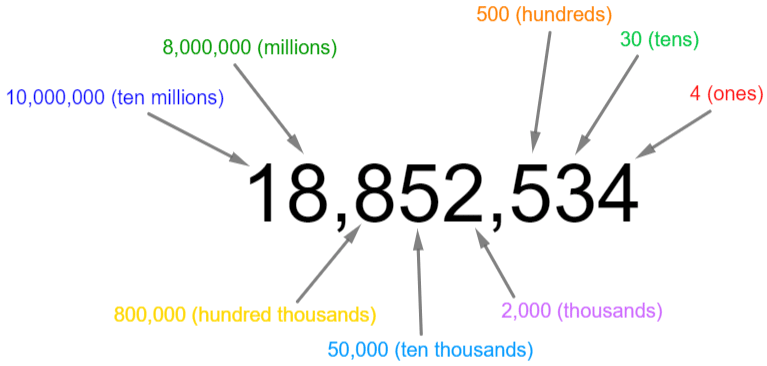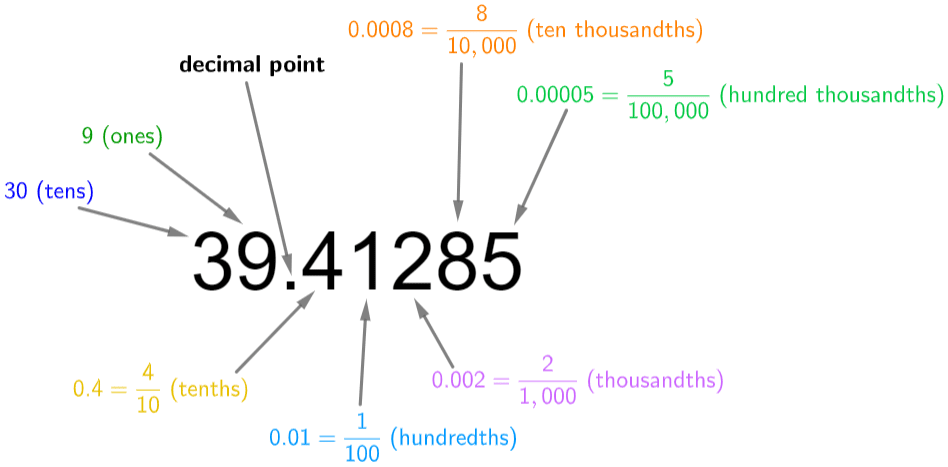# Place Value

GCSEKS3Level 1-3AQACambridge iGCSEEdexcelEdexcel iGCSEOCRWJEC

## Place Value

Place value is all about the value of each digit within a number. Each digit has a different value determined by its position.

Make sure you are happy with the following topics before continuing.

Level 1-3GCSEKS3AQAEdexcelOCRWJECCambridge iGCSEEdexcel iGCSE

## Place Value: Large Numbers

Going from left to right, the value of each digit decreases.Note:  A quick way to state how much a digit is worth is simply to make all the other digits in the number into zeros. This works the same way for decimal place value, as we are about to see.

Level 1-3GCSEKS3AQAEdexcelOCRWJECCambridge iGCSEEdexcel iGCSE

## Place Value: Decimals

decimal is any number with a decimal point. They are the numbers that fall between the integers.

Digits after the decimal place also have a value, but this decreases the further you move away (to the right) from the decimal point.Level 1-3GCSEKS3AQAEdexcelOCRWJECCambridge iGCSEEdexcel iGCSE
Level 1-3GCSEKS3AQAEdexcelOCRWJECCambridge iGCSEEdexcel iGCSE

## Example 1: Large Number

State the place value of the $6$ in $4,609$.

[1 mark]

Counting from right to left, we can see that the $6$ is in the third column along – the hundreds column. Therefore, the value of the ‘$6$ digit’ in this number is $600$.

Level 1-3GCSEKS3AQAEdexcelOCRWJECCambridge iGCSEEdexcel iGCSE

## Example 2: Decimals

In the number $0.56023$, what is

a) The value of the $5$?

b) The value of the $2$?

[2 marks]

a) The $5$ is the first digit after the decimal place, meaning it is in the tenths column. So, it is worth

$\dfrac{5}{10}$ or $0.5$

b) The $2$ is the $4$th digit after the decimal place, meaning it is in the ten thousandths column. So, it is worth

$\dfrac{2}{10,000}$ or $0.0002$

Note: In both of these cases, the trick of “making all other digits into zeros” would give us the correct answer.

Level 1-3GCSEKS3AQAEdexcelOCRWJECCambridge iGCSEEdexcel iGCSE

## Place Value Example Questions

The $8$ is the $3$rd digit from the right, meaning it’s in the hundreds column. So, the value is,

$800$ or $\text{eight hundred}$Gold Standard Education

The $1$ is one place after the decimal point, meaning it is in the tenths column. So, the value is,

$\dfrac{1}{10}$ or $\text{one tenth}$Gold Standard Education

The $6$ is three places after the decimal point, meaning it is in the thousandths column. So, the value is

$\dfrac{6}{1000}$ or $\text{six thousdandths}$Gold Standard Education

The $5$ is the $6$th digit from the right, meaning it’s in the hundred thousands column. So, the value is,

$500,000$ or $\text{five hundred thousand}$Gold Standard Education

$3$ thousands $= 3,000$

$5$ tens $= 50$

$1$ hundredth $= 0.01$

Adding these all together, we get Becky’s number to be

$3,000+50+0.01=3,050.01$Gold Standard EducationGold Standard Education

£19.99 /month. Cancel anytime

Level 1-3GCSEKS3